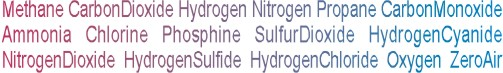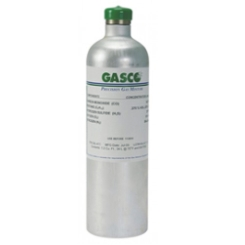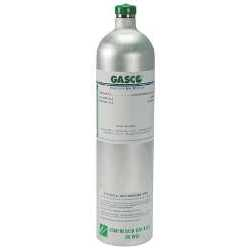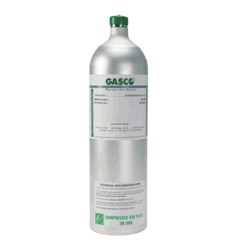Calibration gas blends, mixes and accessories
REACTIVE SINGLE GAS > NITRIC OXIDE :    13 Items found.(1473)

## NORLAB Equivalent  F166050

Norlab Equivalent Calibration Gas 50 ppm Nitric Oxide Balance nitrogen 34L Liter cylinder (Made by Gasco)

Configure

\$178.20(1472)

## NORLAB Equivalent  F166025

Norlab Equivalent Calibration Gas 25 ppm Nitric Oxide Balance nitrogen 34L Liter cylinder (Made by Gasco)

Configure

\$178.20(1471)

## NORLAB Equivalent  F166010

Norlab Equivalent Calibration Gas 10 ppm Nitric Oxide Balance nitrogen 34L Liter cylinder (Made by Gasco)

Configure

\$178.20(1480)

## NORLAB Equivalent  Z1660800

Norlab Equivalent Calibration Gas 800 ppm Nitric Oxide Balance nitrogen 58L Liter cylinder (Made by Gasco)

Configure

\$251.10(1479)

## NORLAB Equivalent  Z1660100

Norlab Equivalent Calibration Gas 100 ppm Nitric Oxide Balance nitrogen 58L Liter cylinder (Made by Gasco)

Configure

\$251.10(1478)

## NORLAB Equivalent  Z166050

Norlab Equivalent Calibration Gas 50 ppm Nitric Oxide Balance nitrogen 58L Liter cylinder (Made by Gasco)

Configure

\$251.10(1477)

## NORLAB Equivalent  Z166030

Norlab Equivalent Calibration Gas 30 ppm Nitric Oxide Balance nitrogen 58L Liter cylinder (Made by Gasco)

Configure

\$251.10(1476)

## NORLAB Equivalent  Z166025

Norlab Equivalent Calibration Gas 25 ppm Nitric Oxide Balance nitrogen 58L Liter cylinder (Made by Gasco)

Configure

\$251.10(1475)

## NORLAB Equivalent  Z166010

Norlab Equivalent Calibration Gas 10 ppm Nitric Oxide Balance nitrogen 58L Liter cylinder (Made by Gasco)

Configure

\$251.10(1474)

## NORLAB Equivalent  Z16605

Norlab Equivalent Calibration Gas 5 ppm Nitric Oxide Balance nitrogen 58L Liter cylinder (Made by Gasco)

Configure

\$251.10(1483)

## NORLAB Equivalent  X166050

Norlab Equivalent Calibration Gas 50 ppm Nitric Oxide Balance nitrogen 74L Liter cylinder (Made by Gasco)

Configure

\$324.00(1482)

## NORLAB Equivalent  X166025

Norlab Equivalent Calibration Gas 25 ppm Nitric Oxide Balance nitrogen 74L Liter cylinder (Made by Gasco)

Configure

\$324.00(1481)

## NORLAB Equivalent  X166010

Norlab Equivalent Calibration Gas 10 ppm Nitric Oxide Balance nitrogen 74L Liter cylinder (Made by Gasco)

Configure

\$324.00